# Fence

The square garden has an area of 537 m2. How many meters is netting necessary to fence the garden?

x =  92.693 m

### Step-by-step explanation: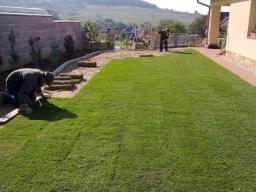Did you find an error or inaccuracy? Feel free to write us. Thank you!#### You need to know the following knowledge to solve this word math problem:

We encourage you to watch this tutorial video on this math problem:

## Related math problems and questions:

• Garden plotCalculate how many meters of fence need to fence the square garden with length and width of 12 meters.
• Fence of the garden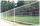The garden has dimensions of 5m and 400cm. How many meters meshes are needed for fencing the plot?
• PlaygroundThe rectangular playground is fenced with 38 m long netting. Its width is 7 m. Calculate its length.
• Perimeter to area 2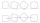Find the area of a square whose perimeter is 100 cm with solve.
• Horses playgroundThe fence for the horses has the shape of a rectangular trapezoid with an area of 400 m2, the base lengths should be 31 m and 19 m. How many meters of boards will they need to fence it if the boards are stacked in 5 rows?
• Perimeter from areaWhat is the perimeter of the square if its content is 64 cm2?
• An equilateral triangle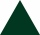The perimeter of an equilateral triangle is 33cm. How long is each side?
• A rectangularA rectangular garden 40 m long and 30 m wide is to be fenced with fence posts at each corner. All the other posts will be 5 meters apart. How many posts will be needed to fence in the garden?
• FloorRectangular floor of living room has a length 5.4 meters and a circumference 17.2 meters. What is its width?
• The schoolyardThe schoolyard had the shape of a square with an 11m side. The yard has been enlarged by 75 m2 and has a square shape again. How many meters was each side of the yard enlarged?
• Rectangular garden 2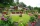A farmer bought 600 m of wire for the fence. He wants to use it to besiege a rectangular garden with a surface of 16875 m2. Calculate the size of the garden.
• GardensThe garden has the square shape with circumference 124 m. Divide it into two rectangular gardens, one should circumference 10 meters more than the second. What size will have a gardens?
• GardenTrapezoid garden has parallel sides 19 m and 24 m. Its area is 193.5 square meters. What is the width of the garden?
• The gardenThe garden has the shape of a rectangular trapezium. The bases have lengths of 27 meters and 36 meters, the trapezoid's height is 12 meters. Calculate how much fence will cost this garden if one meter costs 1.5 €?
• SeedsThe field has a rectangular shape with dimensions of 128 m and 350 m. How many kg of seed are needed for sowing if the 1 m2 will consume 25 g of seeds?
• Rectangular gardenRectangular garden has a length of 48.7 m, a width of 6.3 meters shorter than the length. How much mesh should be bought for its fencing if the gate is 2.9 m long and the gate 1.1 m? What is the area of the garden?
• Office rent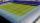For rent 1m2 of office space is paid €165 per year. How much is the annual rent for an office as big as a football field with dimensions 71.5 m and 102.5 m?